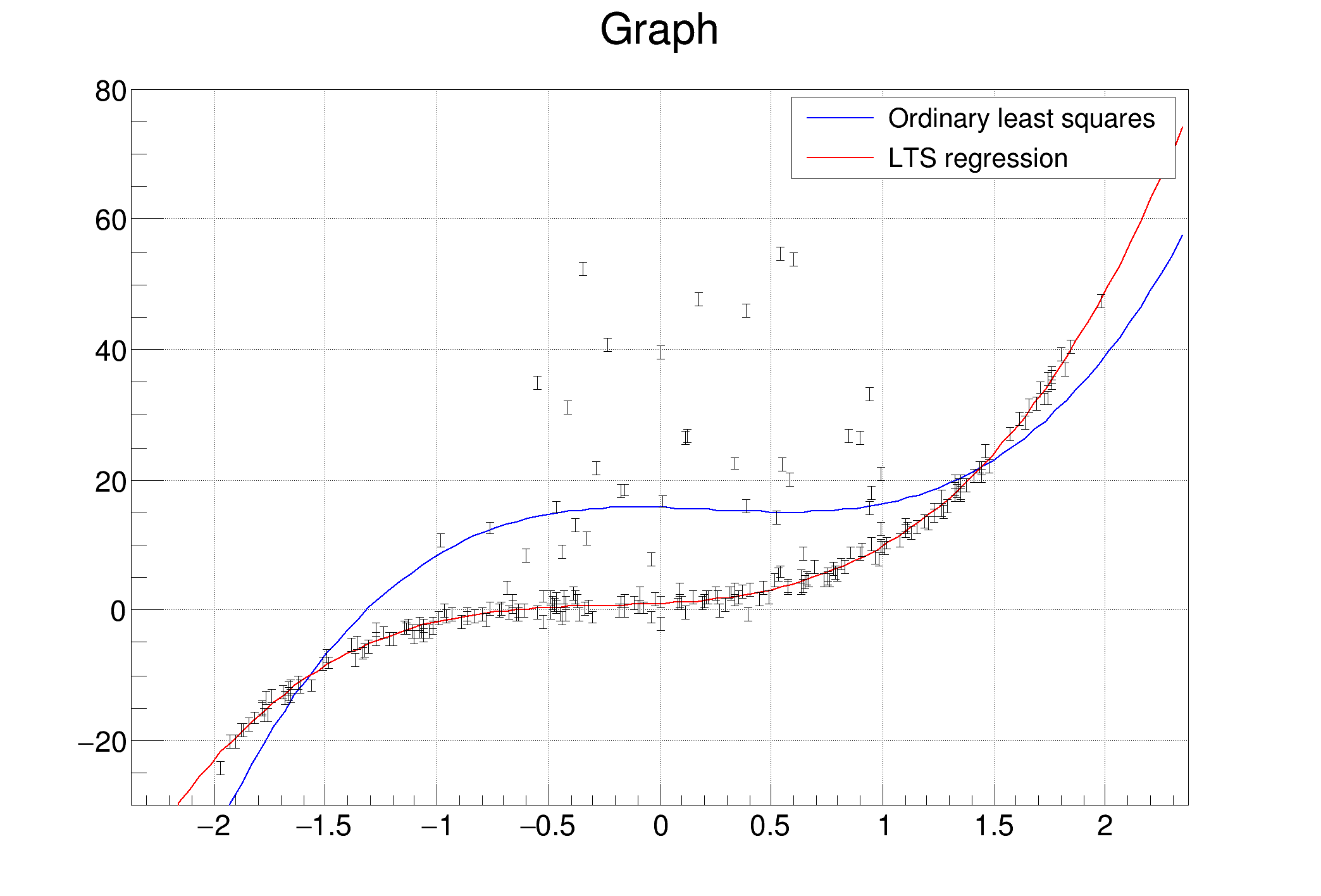ROOT   Reference Guide
Searching...
No Matches
fitLinearRobust.C File Reference

## Detailed DescriptionThis tutorial shows how the least trimmed squares regression, included in the TLinearFitter class, can be used for fitting in cases when the data contains outliers.

Here the fitting is done via the TGraph::Fit function with option "rob": If you want to use the linear fitter directly for computing the robust fitting coefficients, just use the TLinearFitter::EvalRobust function instead of TLinearFitter::EvalOrdinary least squares:
****************************************
Chi2 = 606758
NDf = 246
p0 = 15.724 +/- 0.0887641
p1 = -0.835912 +/- 0.14096
p2 = -3.40616 +/- 0.0607296
p3 = 4.82569 +/- 0.0602628
Resistant Least trimmed squares fit:
****************************************
Minimizer is Linear / Robust (h=0.75)
Chi2 = 634792
NDf = 246
p0 = 1.00953
p1 = 1.71148
p2 = 2.97937
p3 = 4.07752
#include "TRandom.h"
#include "TGraphErrors.h"
#include "TF1.h"
#include "TCanvas.h"
#include "TLegend.h"
void fitLinearRobust()
{
//First generate a dataset, where 20% of points are spoiled by large
//errors
Int_t npoints = 250;
Int_t fraction = Int_t(0.8*npoints);
Double_t *x = new Double_t[npoints];
Double_t *y = new Double_t[npoints];
Double_t *e = new Double_t[npoints];
Int_t i;
for (i=0; i<fraction; i++){
//the good part of the sample
x[i]=r.Uniform(-2, 2);
e[i]=1;
y[i]=1 + 2*x[i] + 3*x[i]*x[i] + 4*x[i]*x[i]*x[i] + e[i]*r.Gaus();
}
for (i=fraction; i<npoints; i++){
//the bad part of the sample
x[i]=r.Uniform(-1, 1);
e[i]=1;
y[i] = 1 + 2*x[i] + 3*x[i]*x[i] + 4*x[i]*x[i]*x[i] + r.Landau(10, 5);
}
TGraphErrors *grr = new TGraphErrors(npoints, x, y, 0, e);
grr->SetMinimum(-30);
grr->SetMaximum(80);
TF1 *ffit1 = new TF1("ffit1", "pol3", -5, 5);
TF1 *ffit2 = new TF1("ffit2", "pol3", -5, 5);
ffit2->SetLineColor(kRed);
TCanvas *myc = new TCanvas("myc", "Linear and robust linear fitting");
myc->SetGrid();
grr->Draw("ap");
//first, let's try to see the result sof ordinary least-squares fit:
printf("Ordinary least squares:\n");
grr->Fit(ffit1);
//the fitted function doesn't really follow the pattern of the data
//and the coefficients are far from the real ones
printf("Resistant Least trimmed squares fit:\n");
//Now let's try the resistant regression
//The option "rob=0.75" means that we want to use robust fitting and
//we know that at least 75% of data is good points (at least 50% of points
//should be good to use this algorithm). If you don't specify any number
//and just use "rob" for the option, default value of (npoints+nparameters+1)/2
//will be taken
grr->Fit(ffit2, "+rob=0.75");
//
TLegend *leg = new TLegend(0.6, 0.8, 0.89, 0.89);
leg->Draw();
delete [] x;
delete [] y;
delete [] e;
}
ROOT::R::TRInterface & r
Definition Object.C:4
#define e(i)
Definition RSha256.hxx:103
int Int_t
Definition RtypesCore.h:45
double Double_t
Definition RtypesCore.h:59
@ kRed
Definition Rtypes.h:66
@ kBlue
Definition Rtypes.h:66
virtual void SetLineColor(Color_t lcolor)
Set the line color.
Definition TAttLine.h:40
The Canvas class.
Definition TCanvas.h:23
1-Dim function class
Definition TF1.h:213
A TGraphErrors is a TGraph with error bars.
virtual void SetMaximum(Double_t maximum=-1111)
Set the maximum of the graph.
Definition TGraph.cxx:2266
virtual TFitResultPtr Fit(const char *formula, Option_t *option="", Option_t *goption="", Axis_t xmin=0, Axis_t xmax=0)
Fit this graph with function with name fname.
Definition TGraph.cxx:1073
virtual void Draw(Option_t *chopt="")
Draw this graph with its current attributes.
Definition TGraph.cxx:769
virtual void SetMinimum(Double_t minimum=-1111)
Set the minimum of the graph.
Definition TGraph.cxx:2275
This class displays a legend box (TPaveText) containing several legend entries.
Definition TLegend.h:23
void SetGrid(Int_t valuex=1, Int_t valuey=1) override